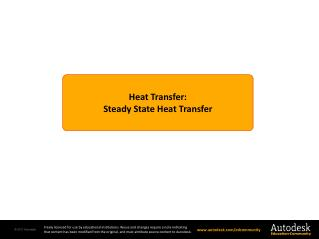DownloadDownload PresentationHeat Transfer : Steady State Heat Transfer

# Heat Transfer : Steady State Heat Transfer

Download Presentation## Heat Transfer : Steady State Heat Transfer

- - - - - - - - - - - - - - - - - - - - - - - - - - - E N D - - - - - - - - - - - - - - - - - - - - - - - - - - -
##### Presentation Transcript

1. HeatTransfer: Steady State Heat Transfer

2. Objectives Section 6 – Thermal Analysis Module4: Steady State Heat Transfer Page 2 • Understand steady state heat transfer. • Explore linear steady state analysis. • Explore nonlinear steady state analysis. • Study an example: • Thermal analysis of a heat sink assembly

3. Steady State Heat Transfer Section 6 – Thermal Analysis Module4: Steady State Heat Transfer Page 3 • Steady state heat transfer occurs when temperatures, thermo-physical properties, surface properties and bulk motion of fluid are constant over time. • Temperature has reached equilibrium. Element under study Heat In Heat Out Any machinery running at constant speed is a good example of steady state heat transfer.

4. Section 6 – Thermal Analysis Module4: Steady State Heat Transfer Page 4 Linear Steady State Heat Transfer • A linear steady state problem is approximated when the thermophysical properties (e.g., conductivity, density, viscosity) are not dependent on temperature. Moreover, radiation loss is unaccounted for. • Thermal conductivity of a material in real life can vary significantly depending upon the material temperature. • If the material properties are fixed, the problem is said to be linear steady state. • If “k” in the above relationship is approximated as not dependent on temperature, then the problem is linear steady state. • An example where a linear steady state heat transfer approximation can be used is heat loss from domestic hot water pipes when the temperature differential is low.

5. Nonlinear Steady State Heat Transfer Section 6 – Thermal Analysis Module4: Steady State Heat Transfer Page 5 • Nonlinear steady state heat transfer occurs when thermophysical properties are assumed to vary with temperature. • This is closer to real life conditions; however more complex to solve. • To solve such problems, an iterative scheme is used by assuming initial temperatures and evaluating through calculations later. • The material properties are adjusted for new temperatures and calculated again until convergence is reached (for example, when heat entering and heat exiting the system boundaries are equal). • Most examples of heat transfer are nonlinear, such as heat loss from a car engine block (engine temperature varies significantly). If “k” in this equation is a function of temperature f(T), than the problem becomes nonlinear.

6. Simplifying Heat Transfer Analysis Section 6 – Thermal Analysis Module4: Steady State Heat Transfer Page 6 • Problems can be simplified by avoiding the need to calculate for conjugate heat transfer. • For instance at low temperatures (<70⁰ C), radiation may be ignored. • If the material properties do not vary significantly (for example, the percentage change is < 20%), the problem can be assumed to be linear steady state. • Likewise, if the effect of body forces such as gravitational force is overwhelmed by forces responsible for bulk fluid flow, natural convection can be ignored. • Geometrical symmetry, if present, should be used to avoid the need to create a full model.

7. Example: Heat Sink Assembly Section 6 – Thermal Analysis Module4: Steady State Heat Transfer Page 7 20° • A heat sink assembly is a common design element in electronics such as desktop computers, laptops and audio systems. • A two-part video presentation for this module using this example is available. The second part covers steady state heat transfer analysis. B C Fins (Aluminium) Heat Spreader (Copper) 40 Watts Microprocessor (Silicon)

8. Summary Section 6 – Thermal Analysis Module4: Steady State Heat Transfer Page 8 • Steady state heat transfer conditions are said to occur when a system is in equilibrium. • For instance, the engine on a car travelling at a constant speed on a highway would more or less lose a fixed amount of heat every second. • Steady state heat transfer can be linear or nonlinear. • Nearly all real life examples are nonlinear; however, systems can be approximated as linear if temperature variation is insignificant.

9. Summary Section 6 – Thermal Analysis Module4: Steady State Heat Transfer Page 9 • If the temperature variation is high, thermal properties of substances involved in heat transfer can change. • For instance: thermal conductivity, fluid density and viscosity changes due to temperature create a nonlinear system which is much more complicated to solve than a linear system. • If thermophysical properties do not vary significantly, linear analysis approximation should be used to reduce computational expense.